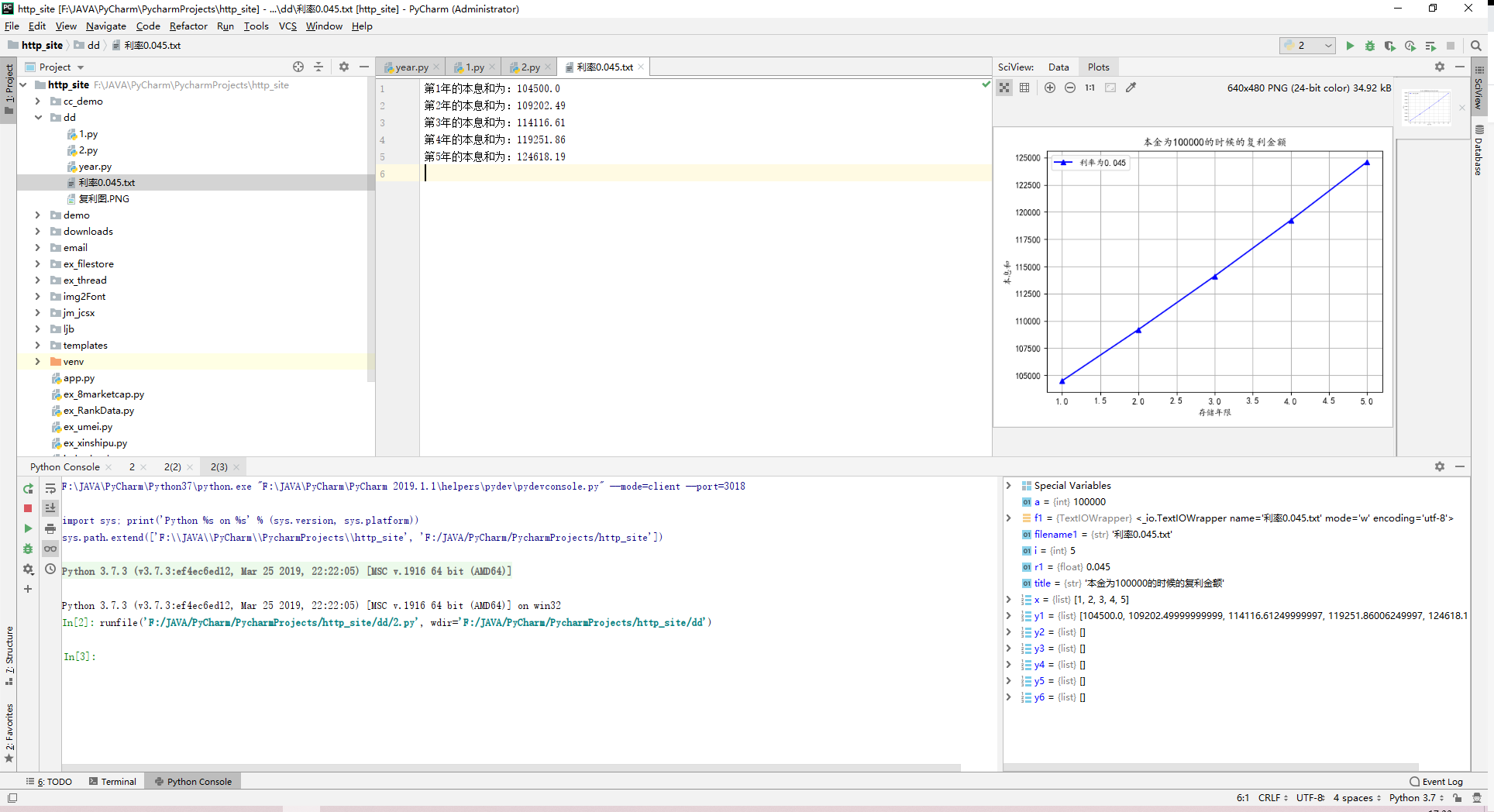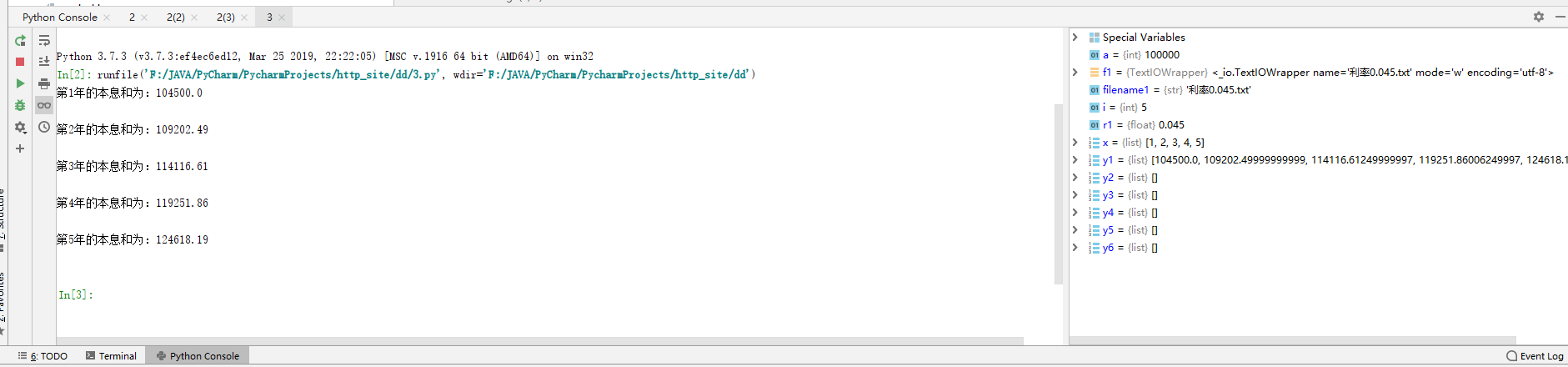2021-06-04 17:21

# python复利计算利息

. 编写一个程序计算10万元本金在银行以4.5%的年利息存5年后得到的收益，按复利计算利息。

• 写回答
• 关注问题
• 收藏
• 邀请回答

#### 2条回答默认 最新

•小P聊技术 2021-06-04 17:33
已采纳
``````# encoding: utf-8
"""
@version: 1.0
@author: AusKa_T
@file: 2
@time: 2021/6/4 0004 17:30
"""
"""
计算复利公式
"""
import matplotlib.pyplot as plt
# 加载字体
import matplotlib as mpl

mpl.rcParams["font.sans-serif"] = ["KaiTi"]
mpl.rcParams["font.serif"] = ["KaiTi"]

a = 100000  # 这里是本金
r1 = 0.045
x = []
y1 = []
y2 = []
y3 = []
y4 = []
y5 = []
y6 = []
# 填入数据
for i in range(1, 6):
x.append(i)
y1.append(a * (1 + r1) ** i)

filename1 = "利率" + str(r1) + ".txt"

with open(filename1, "w", encoding="utf-8") as f1:
for i in range(1, 6):
f1.write(
"第" + str(i) + "年的本息和为：" + str(y1[i - 1]).split('.') + '.' + str(y1[i - 1]).split('.')[:2] + "\n")

# 绘图
title = "本金为" + str(a) + "的时候的复利金额"
plt.title(title)
plt.plot(x, y1, "b^-", label="利率为" + str(r1))
plt.xlabel("存储年限")
plt.ylabel("本息和")

# 显示标签
plt.legend()
# 显示格子
plt.grid()
# 保存图片
plt.savefig("复利图.PNG")
plt.show()``````已采纳该答案
打赏 评论
•小P聊技术 2021-06-04 17:35
``````# encoding: utf-8
"""
@version: 1.0
@author: AusKa_T
@file: 2
@time: 2021/6/4 0004 17:30
"""
"""
计算复利公式
"""
import matplotlib.pyplot as plt
# 加载字体
import matplotlib as mpl

mpl.rcParams["font.sans-serif"] = ["KaiTi"]
mpl.rcParams["font.serif"] = ["KaiTi"]

a = 100000  # 这里是本金
r1 = 0.045
x = []
y1 = []
y2 = []
y3 = []
y4 = []
y5 = []
y6 = []
# 填入数据
for i in range(1, 6):
x.append(i)
y1.append(a * (1 + r1) ** i)

filename1 = "利率" + str(r1) + ".txt"

with open(filename1, "w", encoding="utf-8") as f1:
for i in range(1, 6):
print(
"第" + str(i) + "年的本息和为：" + str(y1[i - 1]).split('.') + '.' + str(y1[i - 1]).split('.')[:2] + "\n")

``````1 打赏 评论